# 人工智能自动规划 03：搜索算法 (2)

## 墨尔本大学 COMP90054 课程笔记

Posted by YEY on March 12, 2020

# Lecture 03 搜索算法 (2)

## 3. 启发函数

### 3.1 系统算法和局部算法

$\to$ 在经典规划领域，启发式搜索算法是最常见，并且几乎也是最成功的搜索算法。

• 贪婪最佳优先搜索（Greedy best-ﬁrst search）
$\to$ 满意规划（satisﬁcing planning）中最流行的 3 种算法之一。
• 加权 $\rm{A}^*$
$\to$ 满意规划（satisﬁcing planning）中最流行的 3 种算法之一。
• $\rm{A}^*$
$\to$ 最优规划（optimal planning）中最流行的算法。（在满意规划中很少使用。）
• 还有很多，诸如 $\rm{IDA}^*$、深度优先分支定界搜索（depth-ﬁrst branch-and-bound search）、宽度优先启发式搜索（breadth-ﬁrst heuristic search）等等。

• 爬山算法（Hill-climbing）
• 增强爬山算法（Enforced hill-climbing）
$\to$ 满意规划（satisﬁcing planning）中最流行的 3 种算法之一。
• 还有很多，诸如 波束搜索（Beam search）、禁忌搜索（tabu search）、遗传算法（genetic algorithms）、模拟退火（simulated annealing）等等。

### 3.2 基本思想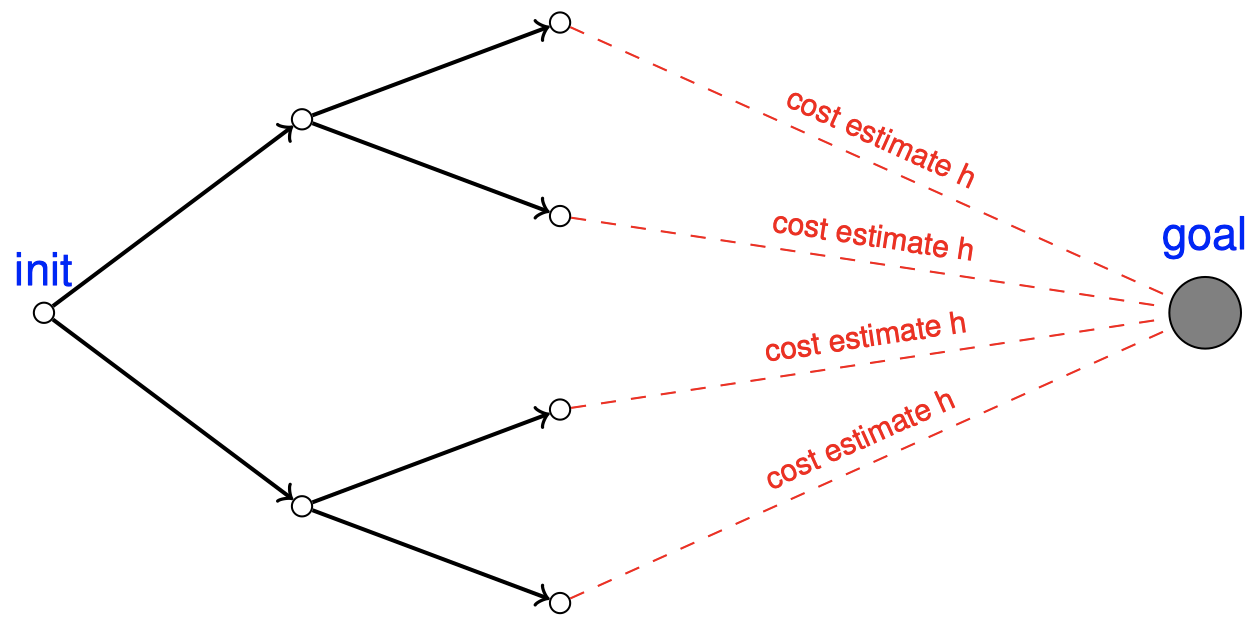$\to$ 启发函数（Heuristic function） $h$ 估计了到达目标的一条最优路径的代价；搜索会倾向于探索具有较小 $h$ 的状态。

### 3.4 关于启发函数的讨论

• 对于很多启发式搜索算法，$h$ 不需要具备任何能够让算法 “工作”（即正确或者完备）的性质。
$\to$ $h$ 可以是任何将状态映射到数字的函数。
• 搜索 性能 关键取决于 “$h$ 对于 $h^*$ 的反映程度有多好”。
$\to$ 这可以被非正式地称为 $h$ 的 信息性（informedness）或者 量（quality）
• 对于某些搜索算法，例如 $\rm{A}^*$，我们可以证明 $h$ 的正式的量性质（formal quality properties）和搜索效率（主要是结点扩展的次数）之间的关系。
• 对于其他的搜索算法，“它在实践中效果很好” 通常是一种很好的分析方法。

$\to$ 我们将详细分析规划中的一个特别重要的启发函数 $h^+$ 的近似值。

“搜索性能的关键取决于 $h$ 的信息性。”

“还有计算 $h$ 时的额外计算开销。”

• $h=h^*$：完美的信息性；计算它 $=$ 在第一步就解决规划问题。
• $h=0$：没有任何信息；可以在常数项时间内被 “计算” 出来。

$\to$ 成功的启发式搜索需要在 $h$ 的信息性和计算它所产生的额外计算开销之间寻找一个好的平衡点。

$\to$ 这就是有关搜索的全部内容的本质。设计方法，以合理的计算成本得出良好的估计。

### 3.5 启发函数的性质

• 安全性（safe）：如果对于所有满足 $h(s)=\infty$ 的 $s\in S$，都有 $h^*(s)=\infty$；

如果启发函数认为当前状态 $s$ 到达目标状态的代价为 $\infty$，即不存在一条到达目标状态的路径，那么 $h^*(s)=\infty$ 则保证了事实上确实不存在这样一条路径。

• 目标察觉性（goal-aware）：如果对于所有的目标状态 $s\in S^G$，都有 $h(s)=0$；

如果启发函数认为当前状态到目标状态的代价为 $0$，那么当前状态应该已经是目标状态了。

• 可采纳性（admissible）：如果对于所有的 $s\in S$，都有 $h(s)\le h^*(s)$；

启发函数估计的从当前状态到目标状态的代价总是小于或者等于实际到达目标状态所需的代价。这意味着我们总是希望启发函数是乐观的，总是低估到达目标状态所需的代价。

• 一致性（consistent）：如果对于所有的状态转移 $s\xrightarrow{\,a\,} s’$，都有 $h(s)\le h(s’)+c(a)$。

如果一致性条件成立，那么意味着任何行动 $a$ 产生的状态转移所导致的启发值的减小量 $h(s)-h(s’)$ 都不会超过这次行动的代价 $c(a)$

$\to$ 彼此之间的关系？

## 4. 系统启发式搜索算法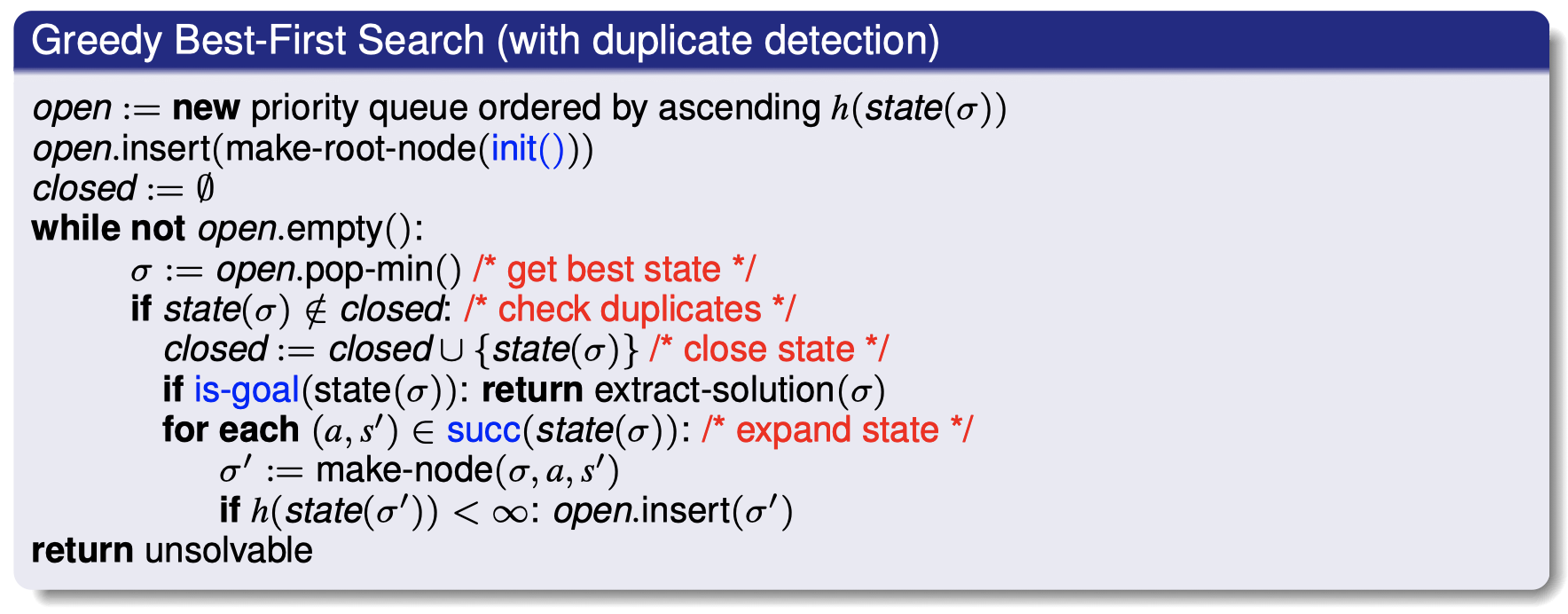• 性质
• 完整性 可以保证吗？
可以，对于具备安全性的启发。（并且重复检测可以避免图中存在环的情况）
• 最优性 可以保证吗？
不能，即使对于完美启发也是。例如：起始状态到目标状态之间存在两种状态转移方式，一个需要付出一百万元的代价，而另一个是免费的。没有什么可以防止贪婪最佳优先搜索选择更差的那个。

可以看到，刚开始，算法探索了左边和上边两个方向，因为它们到目标的距离是一样的。但是当算法探索到上方接近边界处时，发现了右边方向有一条接近目标的路径，最终找到了视频中所示的路径，但是，这条路径显然不是最优的。可以看到，从左边出发然后向下再向右的方向还存在一条更短的路径，但是贪婪最佳优先搜索算法并没有给出。

• 在 $h$ 的所有严格单调变换下不变（例如，使用一个正的常数缩放或着增加一个常数）。
• 实现
• 优先队列：例如一个 最小堆（min heap）
• “检查重复”：可能已经在 “展开状态” 中探索过了；之所以在 “找到当前最优状态”（即将优先队列中距离目标最近的结点 pop 出队列）之后进行此操作只是为了更清楚地指出与 $\rm{A}^*$ 算法的关系。

### 4.2 $\rm{A}^*$ 算法

$\rm{A}^*$ 算法的思想是：相比优先扩展距离目标状态最近的结点（即贪婪最佳优先搜索中具有最小启发值 $h(\rm{state}(\sigma))$ 的结点），我们增加一项，用 $g(\mathrm{state(\sigma)})+h(\mathrm{state}(\sigma))$ 来衡量优先级。其中，启发值 $h(\rm{state}(\sigma))$ 估计了当前结点到达目标结点的代价，而 $g(\mathrm{state(\sigma)})$ 则代表了从起始结点到达当前结点的代价，二者之和越小，则对应的结点扩展优先级越高。

• 我们从初始结点 $I$ 开始，它的 $g(\mathrm{state}(I))=0$ 因为是起始结点，启发值 $h(\mathrm{state}(I))=3$。然后，我们有一个目标结点 $\gamma$。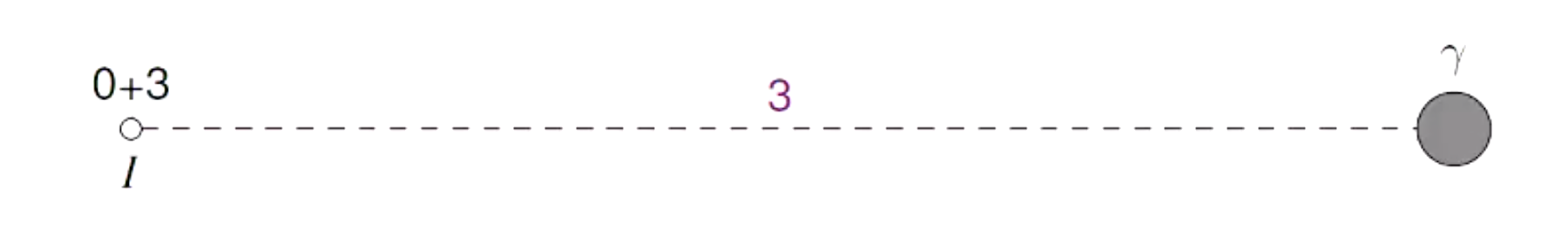• 我们对结点 $I$ 进行扩展，得到两个后继结点，它们对应的 $g(\mathrm{state(\sigma)})+h(\mathrm{state}(\sigma))$ 分别为 $(1+2)$ 和 $(1+3)$。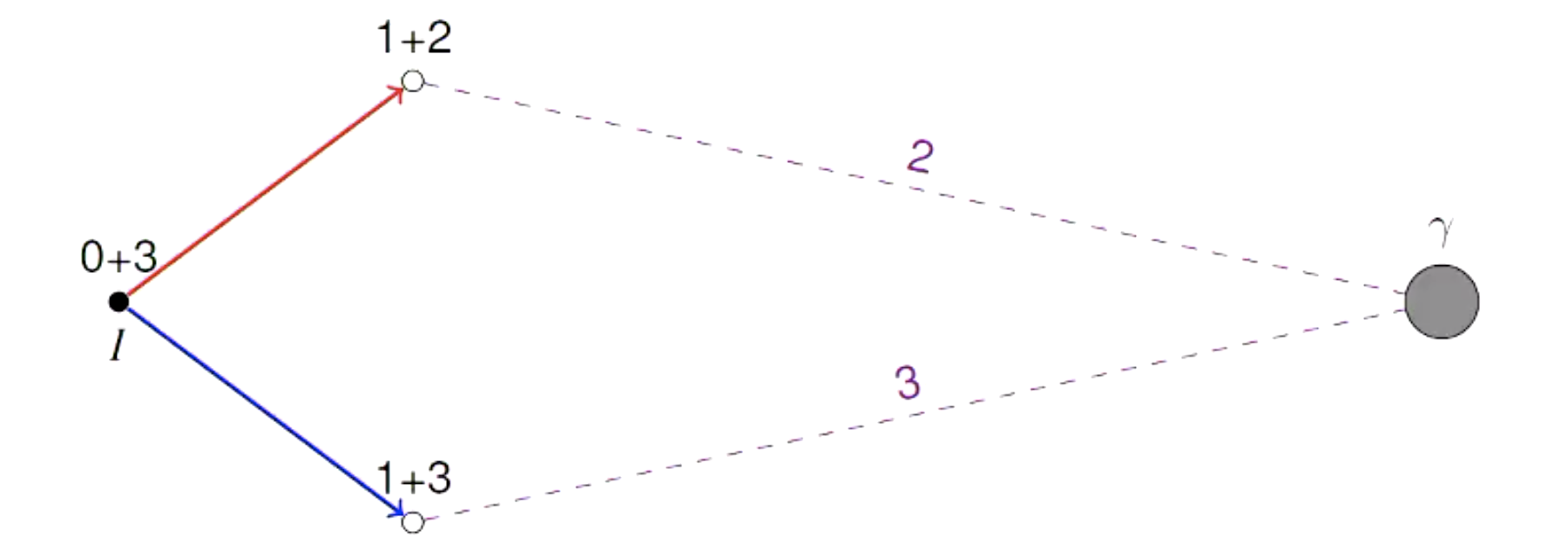• 我们选择 $(1+2)$ 对应的结点继续进行扩展，得到 $(2+6)$ 和 $(2+7)$ 对应的两个后继结点。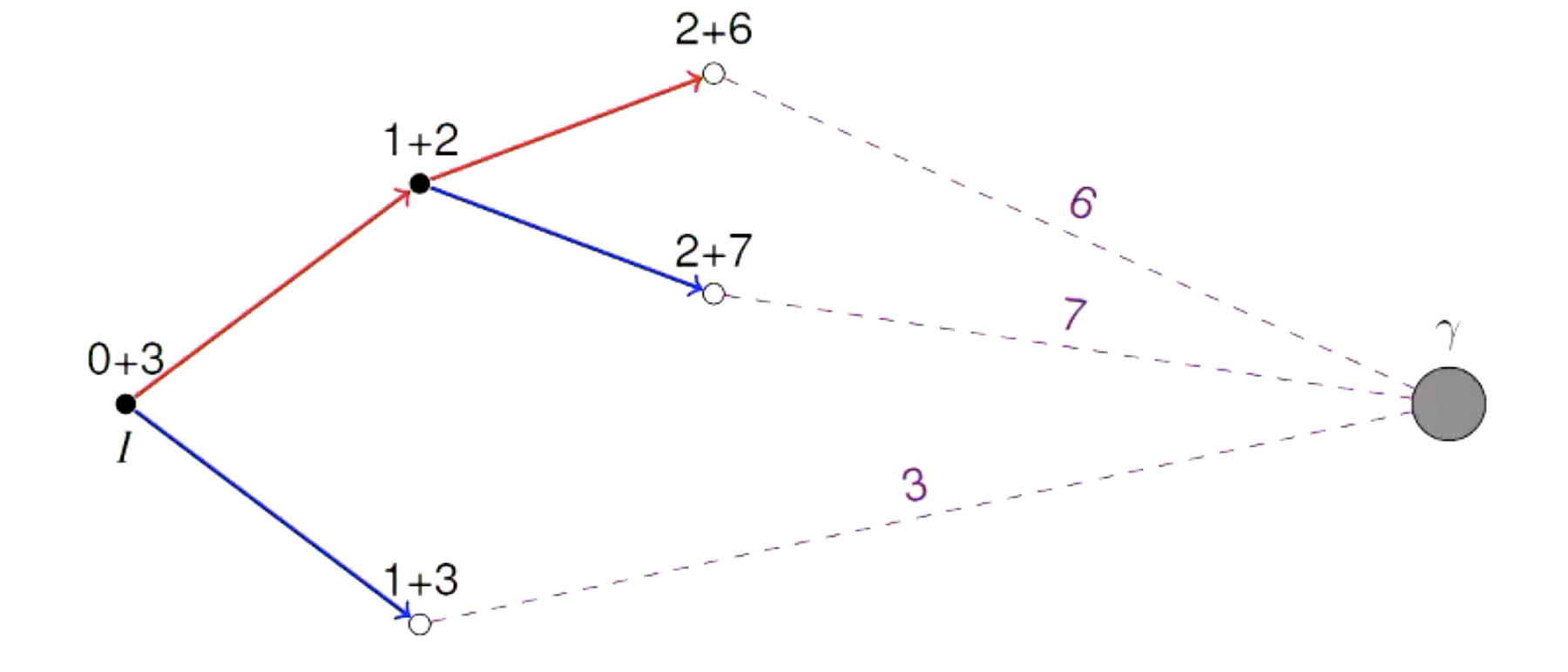• 此时，我们的优先队列中一共存在 3 个结点：$(1+3)$、$(2+6)$ 和 $(2+7)$，因此，接下来我们选择 $(1+3)$ 对应的结点进行扩展，并将后继结点 $(2+5)$ 和 $(2+2)$ 放入优先队列中。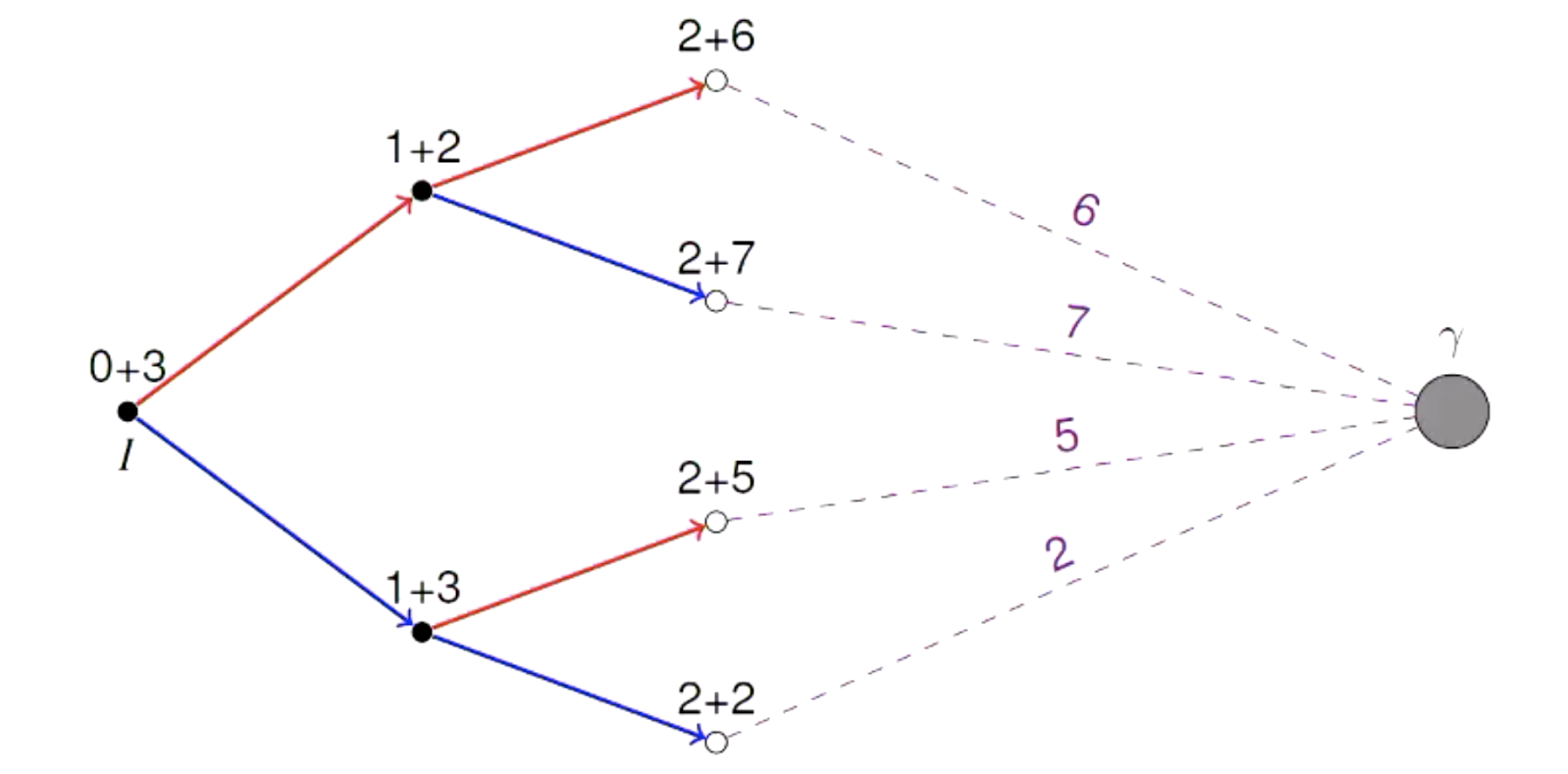• 此时，优先队列中一共存在 4 个结点：$(2+6)$、$(2+7)$、$(2+5)$ 和 $(2+2)$，我们选择结点 $(2+2)$ 进行扩展，得到 $(3+5)$ 和 $(3+1)$，继续将这两个后继结点加入优先队列中。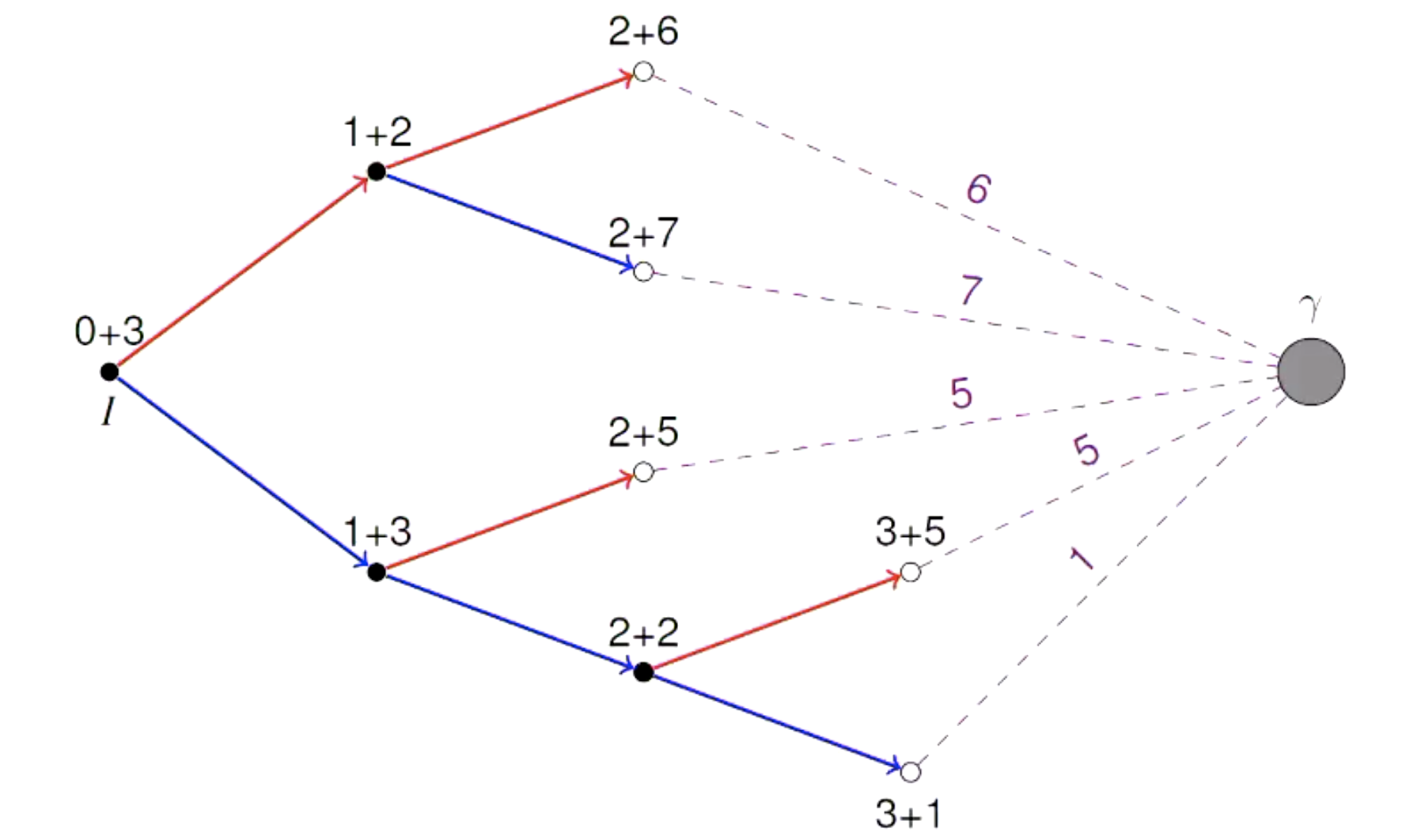• 此时，优先队列中一共存在 5 个结点：$(2+6)$、$(2+7)$、$(2+5)$、$(3+5)$ 和 $(3+1)$，我们选择结点 $(3+1)$ 进行扩展，得到 $\gamma$ 和 $(4+8)$。至此，我们已经找到了目标结点 $\gamma$，并且得到了一条代价为 $4$ 的最优路径：$I\to (1+3) \to (2+2) \to (3+1) \to \gamma$。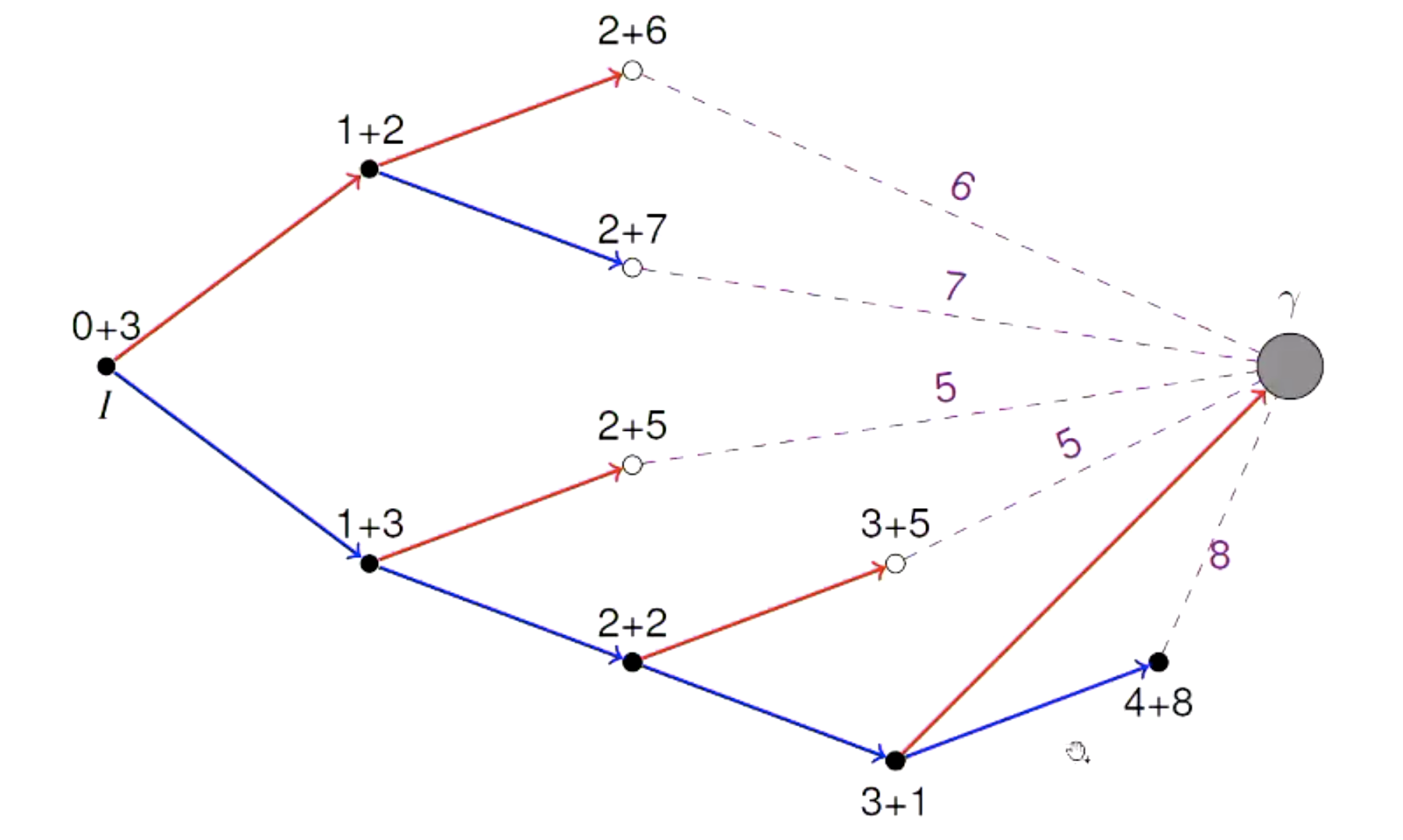$\rm{A}^*$ 算法相关术语

• 一个状态的 $f$ 值：定义为 $f(s):=g(s)+h(s)$
正如之前提到的，$g(s)$ 衡量了起始状态到当前状态的代价，$h(s)$ 则估计了当前状态到目标状态的代价，两者之和 $f(s)$ 则估计了这条搜索路径的代价。$f$ 值越小，对应结点在优先队列中的扩展优先级越高。
• 生成结点（Generated nodes）：在某个位置插入 $\rm{open}$ 的结点（即由当前结点扩展所生成的后继结点）。
• 扩展结点（Expanded nodes）：从 $\rm{open}$ 中 pop 出的结点 $\sigma$，用于根据测试 $\rm{closed}$ 和距离来生成后继结点。
• 重新扩展结点（Re-expanded nodes）：扩展那些状态 $\mathrm{state}(\sigma)\in \mathrm{closed}$ 的结点（又称 重新开放（re-opened）结点）。
所以，在 $\rm{A}^*$ 算法中，那些 $\rm{closed}$ 中的结点是有可能重新加入 $\rm{open}$ 中的。

例如，下图中 $I$ 为起始结点，$G$ 为目标结点：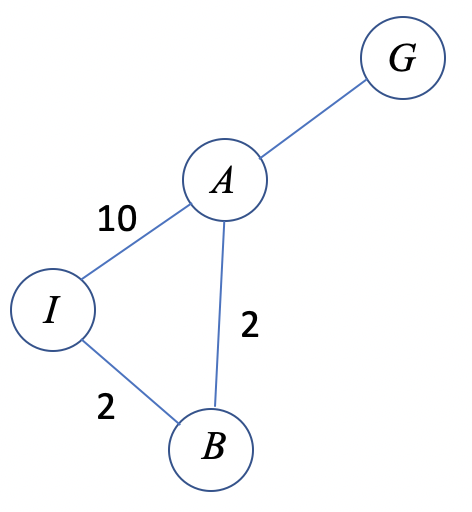当我们扩展结点 $I$ 时，我们会得到 $A$、$B$ 两个后继结点。我们选择 $B$ 结点进行扩展，然后再次得到后继结点 $A$。然后，我们发现同样是从 $I$ 到 $A$：路径 $I\to B\to A$ 的代价要比路径 $I\to A$ 的代价更小。

$\rm{A}^*$ 算法（包含重复检测和重新开放）的伪代码描述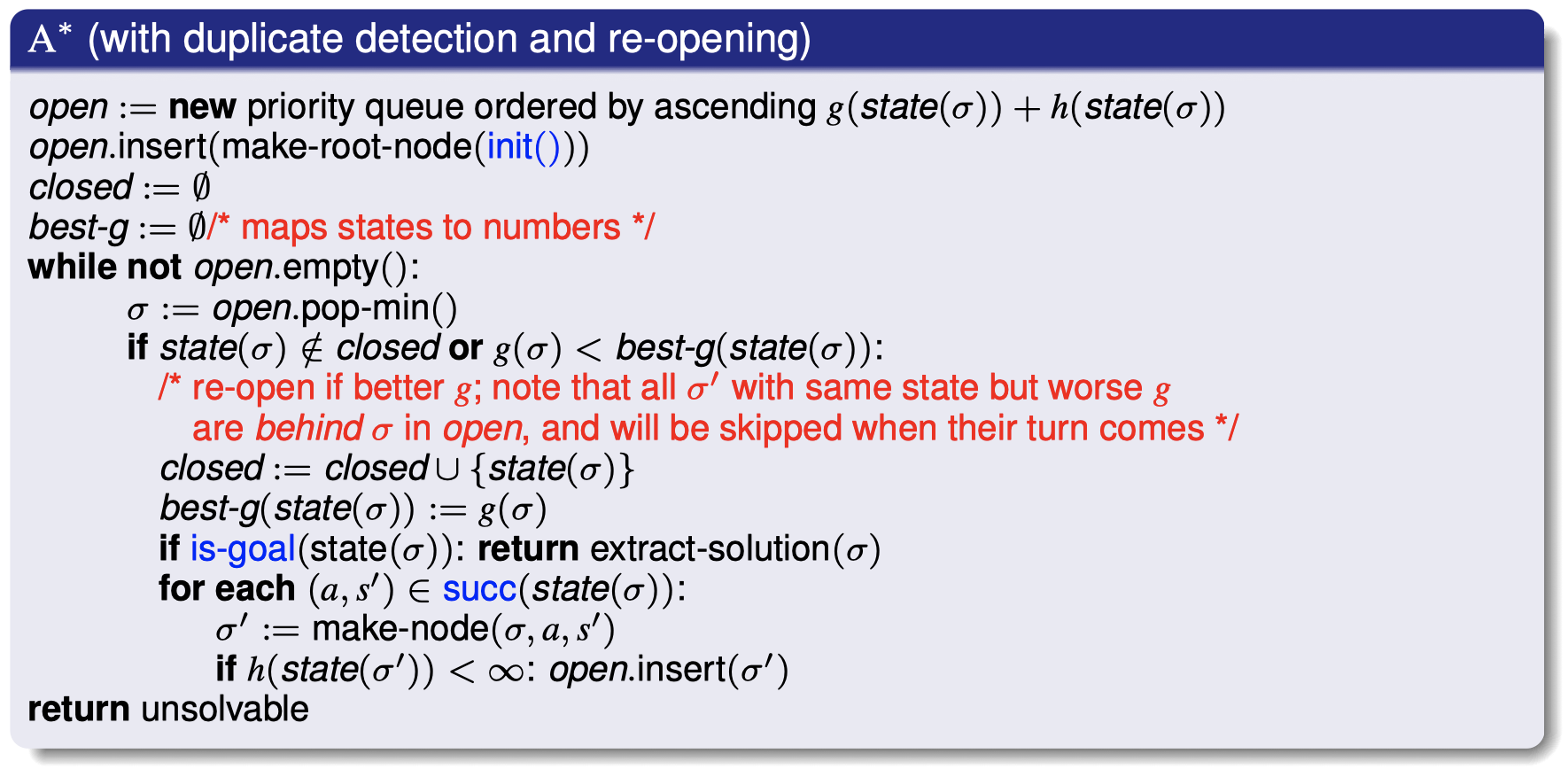$\rm{A}^*$ 算法评估

• 性质
• 完整性 可以保证吗？
可以，对于具备安全性的启发。（即使没有重复检测）
• 最优性 可以保证吗？
可以，对于具备可采纳性的启发。（即使没有重复检测）
• 实现
• 流行方法：对于 $f(s)=f(s’)$ 的情况，选择其中 $h$ 值较小的状态对应的结点进行扩展。
• 如果 $h$ 具备可采纳性和一致性，那么 $\rm{A}^*$ 算法永远不会 re-open 一个 state。所以，如果我们事先知道这一点，可以对算法进行简化。
• 常见的难以察觉的错误：在错误的位置检查重复项。（Russel & Norvig 在《人工智能：一种现代方法》一书中对这一点的描述太不精确了。）
• 上面的伪代码实现是基于可读性而非效率进行了优化。

A. 宽度优先搜索 $\qquad$ B. 深度优先搜索 $\qquad$ C. 一致代价搜索 $\qquad$ D. 深度受限搜索

### 4.3 加权 $\rm{A}^*$ 算法（Weighted $\rm{A}^*$）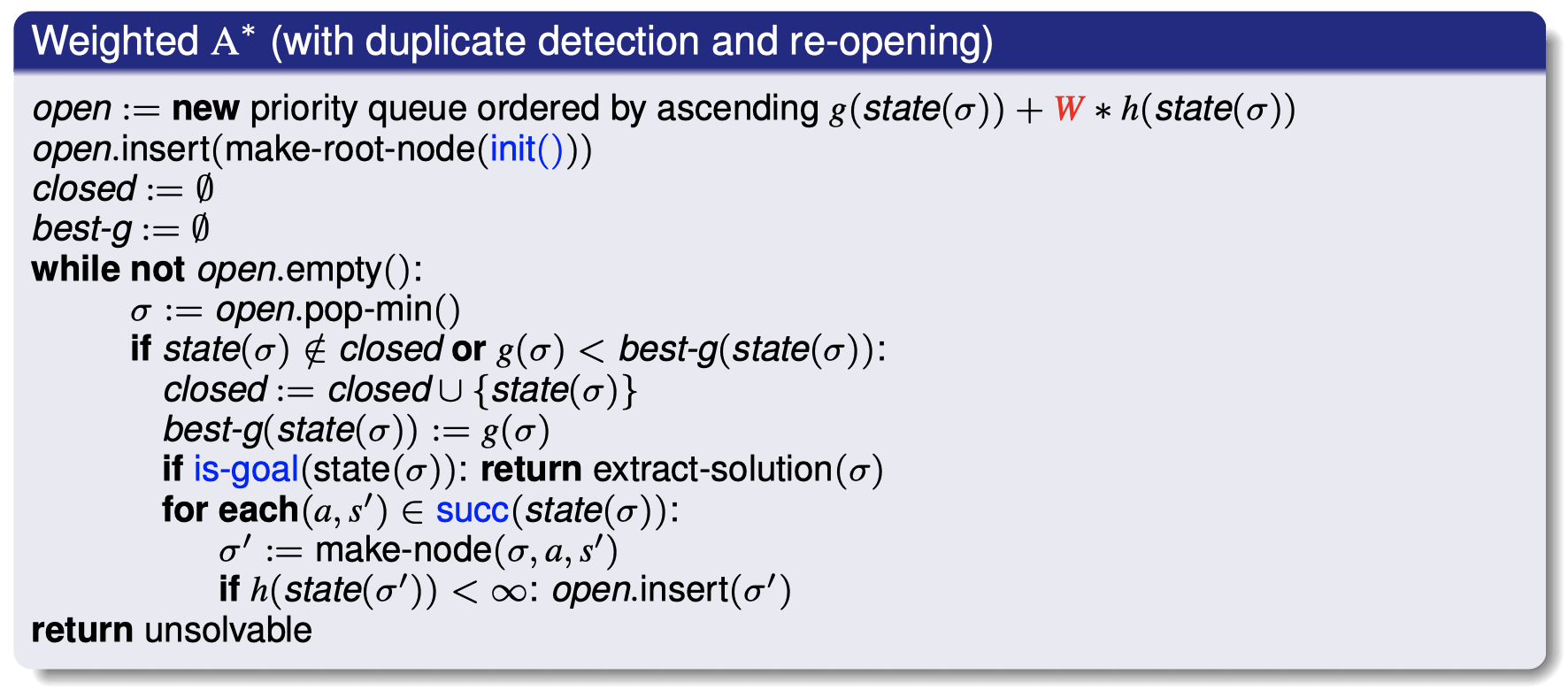• 权重 $W\in \mathbb R_0^+$ 是一个 算法参数

• 对于 $W=0$ 的情况，加权 $\rm{A}^*$ 的行为就像一致代价搜索；
• 对于 $W=1$ 的情况，加权 $\rm{A}^*$ 的行为就像 $\rm{A}^*$；
• 对于 $W\to \infty$ 的情况，加权 $\rm{A}^*$ 的行为就像贪婪最佳优先搜索。
• 性质

• 对于 $W>1$ 的情况，加权 $\rm{A}^*$ 是 有界次优的（bounded suboptimal）：如果 $h$ 具备可采纳性，那么算法返回解的代价不会超过最优解代价的 $W$ 倍。
这个性质在实践中非常强大，这也是加权 $\rm{A}^*$ 算法在实践中如此受欢迎的原因。假设我们的预期的算法搜索时间是半个小时，最优解的时间在半小时以内，那么一种技巧是采用加权 $\rm{A}^*$ 算法，并且将初始 $W$ 设置的非常大，我们将得到一个类似贪婪最佳优先搜索的算法，通常会很快给出一个解（一般会比 $\rm{A}^*$ 算法快很多），然后我们可以逐步降低 $W$ 的值，每次我们都会得到一个具有更小路径代价的解，然后我们可以通过不断这样尝试找到一个次优解。

## 5. 局部启发式搜索算法

### 5.1 爬山算法（Hill-Climbing）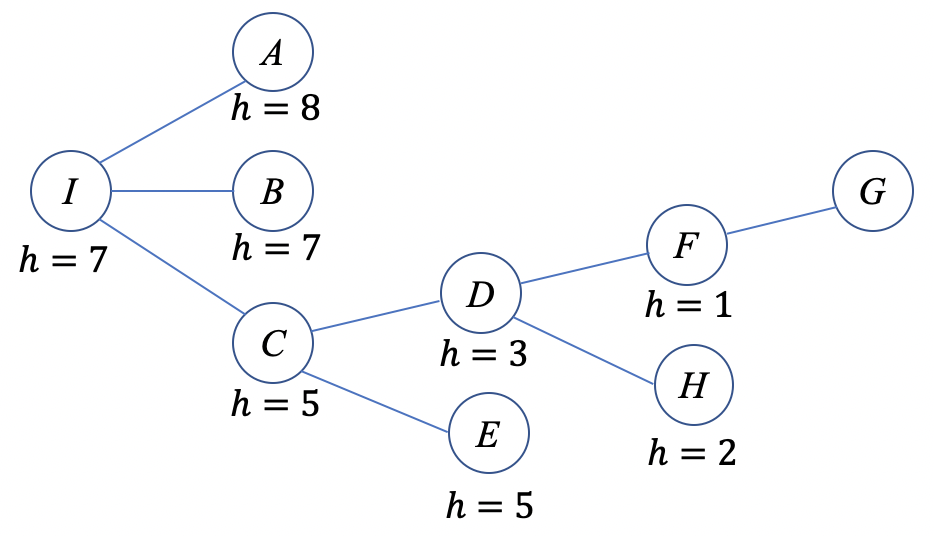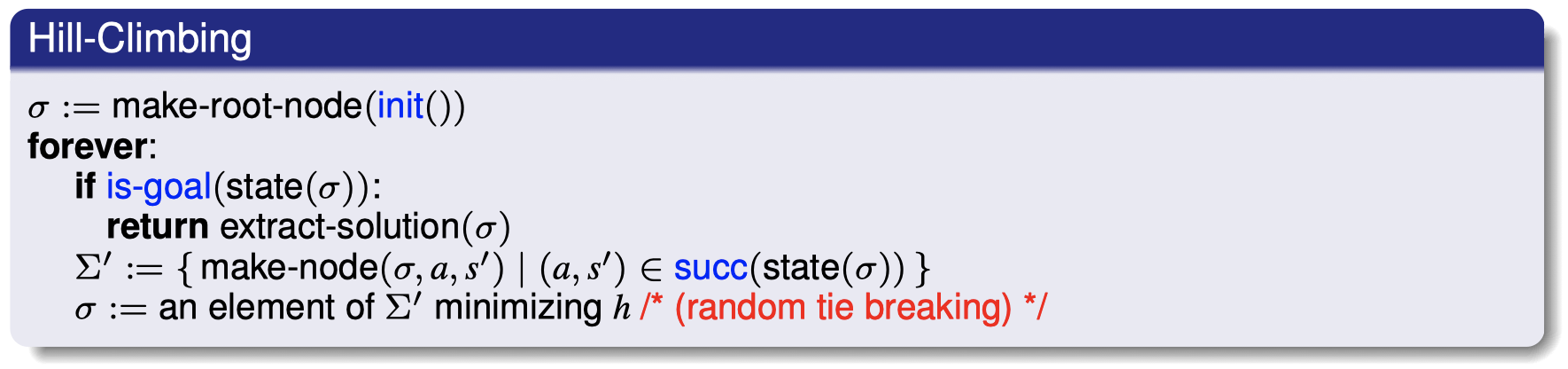• 仅当对于所有 $s\notin S^G$ 都有 $h(s)>0$ 时，算法才有意义。
• 完整性或者最优性可以满足吗？
不能。
• 当 $h(\sigma)$ 无法立即增加时，爬山算法非常容易陷入局部代价最小值点。
如果爬山算法在搜索过程中进入死胡同怎么办？
没有办法。本质上来看，爬山算法可以视为梯度下降的连续版本。梯度下降通常用于寻找图中的最高点或者最低点，所以它经常会陷入局部最优解。
• 很多策略可以处理这种情况：平局决胜制（tie-breaking strategies）、重启（restarts）、……
• 在大部分 AI 规划问题中，我们通常不会直接使用爬山算法，尽管它在很多机器学习问题中应用很普遍。

### 5.2 增强爬山算法（Enforced Hill-Climbing）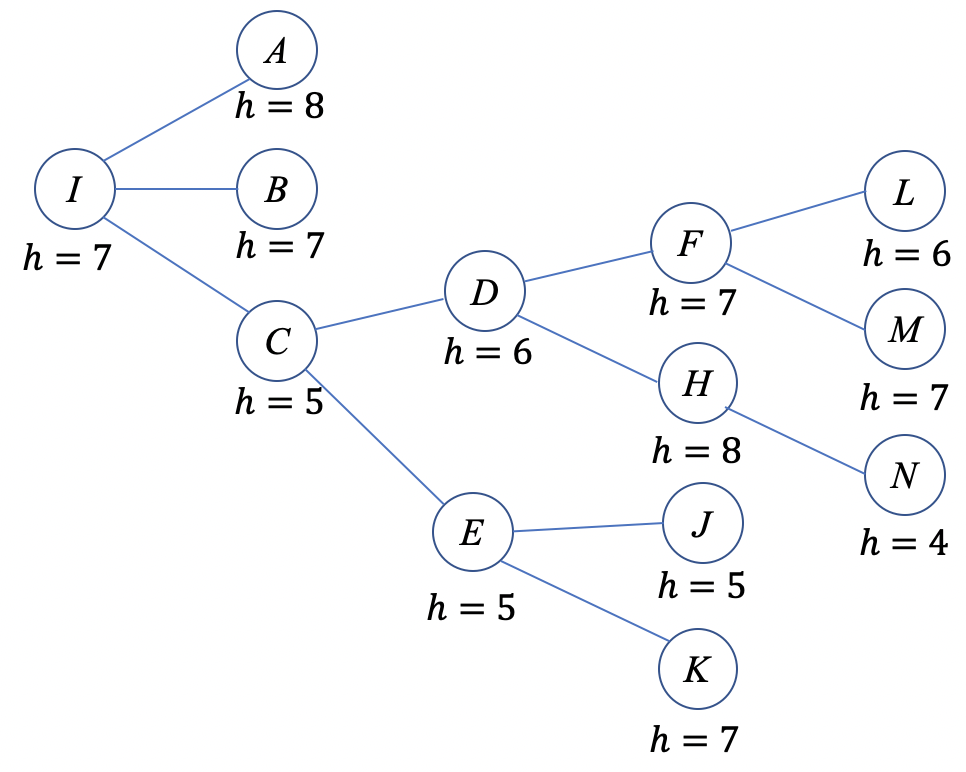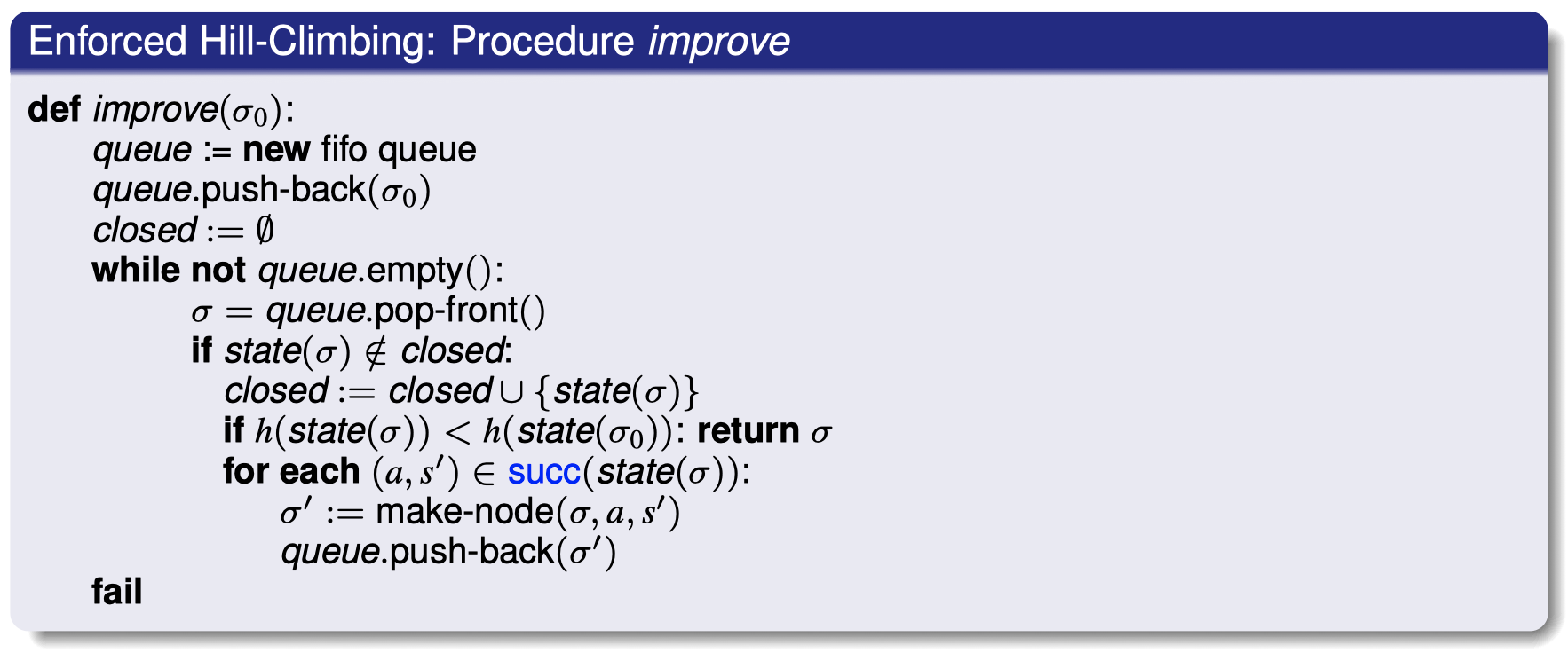$\to$ 在找到具有严格更小 $h$ 值的后继结点之前，采用宽度优先搜索策略。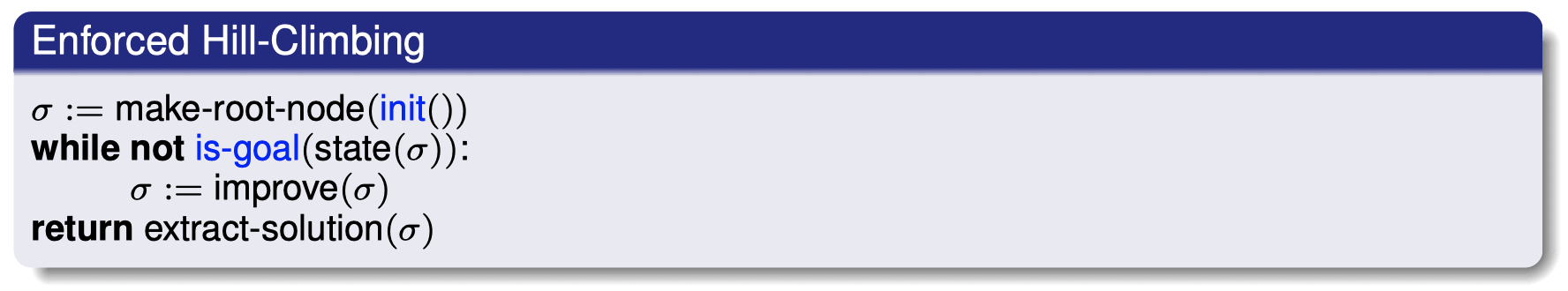• 仅当对于所有 $s\notin S^G$ 都有 $h(s)>0$ 时，算法才有意义。
• 最优性可以满足吗？
不能。它在选择路径时很像贪婪优先搜索，在实际问题中我们不太可能得到最优解。
• 完整性可以满足吗？
通常来说，不能。在特定情况下，可以，假设 $h$ 具备目标察觉性（goal-aware）。
$\to$ 过程改进有可能失败：在所有从状态 $s$ 出发所能够抵达的后继结点中，可能并不存在具备严格更小 $h$ 值的状态。在这种情况下，从状态 $s$ 出发并不能到达（我们假设的）目标状态。
$\to$ 如果状态空间是无向的，那么这种情况就不会发生，即对于状态空间 $\Theta_{\Pi}$ 中的所有可能的状态转移 $s\to s’$，都存在一个对应的状态转移 $s’\to s$。

### 5.3 几种搜索算法的性质对比

DFS BFS ID A* HC IDA*

• 参数：$d$ 是解所在的深度；$b$ 是分支因子
• 宽度优先搜索（BFS）满足最优性的前提是代价一致。
• $\rm{A}^*\,/\,\rm{IDA}^*$ 满足最优性的前提是 $h$ 具备 可采纳性（admissible）：$h< h^*$

## 6. 总结

### 6.1 思考

• 问题 1：如果对于所有结点 $n$，我们都设置 $h(n):=0$，那么 $\rm{A}^*$ 算法会变成什么？

A. 宽度优先搜索 $\qquad$ B. 深度优先搜索 $\qquad$ C. 一致代价搜索 $\qquad$ D. 深度受限搜索

答案：C，两者具有相同的结点扩展顺序。（$\rm{open / closed}$ 状态的细节可能存在一些差异）

• 问题 2：如果对于所有结点 $n$，我们都设置 $h(n):=0$，那么贪婪最佳优先搜索会变成什么？

A. 宽度优先搜索 $\qquad$ B. 深度优先搜索 $\qquad$ C. 一致代价搜索 $\qquad$ D. A、B 和 C

答案：D，我们对 $h$ 的设置使得所有的结点的优先级顺序都不复存在，因此，问题完全取决于我们对于 $\textrm{open list}$ 中的结点采用哪种平局决胜制：A 选项对应 FIFO；B 选项对应 LIFO；C 选项对应将 $g$ 作为优先级标准。（$\rm{open / closed}$ 状态的细节可能存在一些差异）

• 问题 3：有信息搜索总是比盲目搜索更好吗？
答案：不是的。
$\to$ 在贪婪最佳优先搜索中，启发函数可能会导致巨大的搜索空间，可能远超代价一致搜索。例如，在路径规划中，假如你想从墨尔本去往悉尼，但是 $h($珀斯$)<h($堪培拉$)$。
$\to$ 在具有一个可采纳启发函数和重复检查的 $\rm{A}^*$ 搜索中，我们得到的结果不可能比一致代价搜索更差：$h(s)>0$ 只能减少为了证明最优性所必须考虑的状态数。
$\to$ 同样，在上面的例子中，任何具有可采纳启发函数的 $\rm{A}^*$ 算法都不会对 “珀斯” 结点进行展开，因为 $g($珀斯$)>g($悉尼$)$。
$\to$ “信任启发函数” 这种做法有其自身的危险性，有时候，$g$ 可以帮助我们减少搜索次数。

### 6.2 总结

• 世界状态：通过规划任务所建模的世界中的情况。
• 搜索状态：留待解决的子问题。
• 前进 搜索中，世界状态和搜索状态是相同的。
• 后退 搜索中，搜索状态是描述世界状态集的子目标。
• 搜索结点：搜索状态 + 有关 “我们如何到达该状态” 的信息。

• 盲目 vs. 启发式有信息）搜索
• 系统 vs. 局部 搜索

• 通常：信息 越多，表现越好。
• 性质：安全性、目标察觉性、可采纳性、一致性
• 理想情况：完美启发 $h^*$

• 用于 满意规划 的最常见算法：
• 贪婪最佳优先搜索
• 加权 $\rm{A}^*$
• 增强爬山算法
• 用于 最优规划 的最常见算法：
• $\rm{A}^*$

## 7. 扩展阅读

• Artiﬁcial Intelligence: A Modern Approach (Third Edition), Chapter 3 “Solving Problems by Searching” and the ﬁrst half of Chapter 4 “Beyond Classical Search”.
• Content: An overview of various search algorithms, including blind searches as well as greedy best-ﬁrst search and $\rm{A}^*$.
• Search Tutorial in the context of path-ﬁnding http://www.redblobgames.com/pathfinding/a-star/introduction.html本作品采用知识共享署名-非商业性使用-相同方式共享 4.0 国际许可协议进行许可。 欢迎转载，并请注明来自：YEY 的博客 同时保持文章内容的完整和以上声明信息！